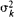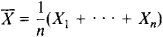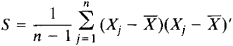# multivariate analysis

Also found in: Dictionary, Thesaurus, Medical, Financial, Wikipedia.

## multivariate analysis

[¦məl·tē′ver·ē·ət ə′nal·ə·səs]
(statistics)
The study of random variables which are multidimensional.
McGraw-Hill Dictionary of Scientific & Technical Terms, 6E, Copyright © 2003 by The McGraw-Hill Companies, Inc.

## multivariate analysis

the analysis of data collected on several different VARIABLES. For example, in a study of housing provision, data may be collected on age, income, family size (the ‘variables’) of the population being studied. In analysing the data the effect of each of these variables can be examined, and also the interaction between them.

There is a wide range of multivariate techniques available but most aim to simplify the data in some way in order to clarify relationships between variables. The choice of method depends on the nature of the data, the type of problem and the objectives of the analysis. FACTOR ANALYSIS and principle component analysis are exploratory, and used to find new underlying variables. CLUSTER ANALYSIS seeks to find natural groupings of objects or individuals. Discriminant analysis is a technique designed to clarify the differentiation between groups influenced by the independent variable(s). Other techniques, e.g. multiple REGRESSION ANALYSIS, aim to explain the variation in one variable by means of the variation in two or more independent variables. MANOVA (multivariate analysis of variance), an extension of the univariate ANALYSIS OF VARIANCE, is used when there are multiple independent variables, as in the example above. An example of multivariate techniques for analysing categorical data is LOG-LINEAR ANALYSIS.

Collins Dictionary of Sociology, 3rd ed. © HarperCollins Publishers 2000
The following article is from The Great Soviet Encyclopedia (1979). It might be outdated or ideologically biased.

## Multivariate Analysis

the branch of mathematical statistics dealing with methods of studying statistical data concerning objects for which more than one quantitative or qualitative characteristic is measured. In the area of multivariate analysis that has been most intensively investigated, it is assumed that the results of individual observations are independent and obey the same multivariate normal distribution. The term “multivariate analysis” is sometimes applied in a narrow sense to this area.

In more precise language, multivariate analysis deals with data where the result Xj of the l th observation can be expressed in terms of the vector Xj = (Xj1, Xj2, . . . , Xjs). Here, the random variable Xjk has the mathematical expectation μk and variance, and the correlation coefficient between Xjk and Xjl is ρkl. Of great importance are the mathematical expectation vector μ = (μl, . . . ,μs) and the covariance matrix Σ with elements σkσlσkl, where k, 1 = 1, . . . , s. This vector and matrix define completely the distribution of the vectors Xl . . . , Xn, which are the results of n independent observations. The choice of the multivariate normal distribution as the principal mathematical model for multivariate analysis can be justified in part by the following considerations: on the one hand, this model can be used in a great number of applications; on the other hand, only within the framework of this model can exact distributions of sample characteristics be calculated. The sample meanand the sample covariance matrixare maximum likelihood estimators of_the corresponding parameters of the population; here, (Xj)′ is the transpose of (Xj) (seeMATRIX). The distribution of is normal (µ,∑/n). The joint distribution of the elements of the covariance matrix S, known as the Wishart distribution, is a natural generalization of the chi-square distribution and plays an important role in multivariate analysis.

A number of problems in multivariate analysis are more or less analogous to the corresponding univariate problems—for example, the problem of testing hypotheses on the equality of the means in two independent samples. Examples of other problems are the testing of hypotheses on the independence of particular groups of components of the vectors Xj and the testing of such special hypotheses as the spherical symmetry of the distribution of the Xj The need to understand the complicated relationships between the components of the random vectors Xj leads to new problems. The method of the principal components and the method of canonical correlations are used to reduce the number of random characteristics—that is, the number of dimensions—under consideration or to reduce the characteristics to independent random variables.

In the method of principal components, the vectors Xj are carried by a transformation into the vectors Yj = (Yjl..... , Yjr). The components of the Yj are chosen such that Yjl has the maximum variance among the normalized linear combinations of the components of X1, Yj2 has the maximum variance among the linear functions of the components of X1 uncorrelated with Yj1, and so on.

In the method of canonical correlations, two sets of random variables (components of Xj) are replaced by smaller sets. First, linear combinations, one from each set of variables, are constructed so as to have maximum simple correlation with each other. These linear combinations are called the first pair of canonical variables, and their correlation is the first canonical correlation. The process is continued with the construction of further pairs of linear combinations. It is required, however, that each new canonical variable be uncorrelated with all previous ones. The method of canonical correlations indicates the maximum correlation between linear functions of two groups of components of the observation vector.

The results of the method of principal components and the method of canonical correlations contribute to an understanding of the structure of the multivariate population under consideration. Also of use in this regard is factor analysis, in which the components of the random vectors Xj are assumed to be linear functions of some unobserved factors that are to be studied.

Multivariate analysis also deals with the problem of differentiating two or more populations from the results of observations. One aspect of this problem is known as the discrimination problem and consists in the assignment of a new element to one of several populations on the basis of an analysis of samples of the populations. Another aspect involves dividing the elements of a population into groups that differ maximally, in some sense, from each other.

### REFERENCES

Anderson, T. Vvedenie v mnogomernyi statisticheskii analiz. Moscow, 1963. (Translated from English.)
Kendall, M. G., and A. Stuart. The Advanced Theory of Statistics, vol. 3. London, 1966.
Dempster, A. P. Elements of Continuous Multivariate Analysis. London, 1969.

A. V. PROKHOROV

References in periodicals archive ?
A concise and thorough introduction to multivariate analysis which provides full concepts and derivations of many methods and techniques, this text is suitable for advanced self-study.
A second multivariate analysis assessed associations between maternal employment changes and low birth weight in infants, taking into account two other factors possibly influencing birth weight (maternal race and prepregnancy weight).
The multivariate analysis examined the contributions of about 20 factors, including age, performance status, site of primary tumor, site of metastases, hematologic parameters, and biochemical parameters.
In a multivariate analysis with adjustment for the conventional stroke risk factors, women with a baseline history of migraine with aura--at any time, not just within the past year--proved to be at a statistically significant 53% increased risk of total stroke and a 70% increased- risk of ischemic stroke, compared with women without a history of headache.
The likelihood of having advanced colorectal neoplasia was increased by 85% with current smoking, by 66% in patients with at least one first-degree relative with colorectal cancer, and by 2% with moderate to heavy current drinking in a multivariate analysis. In contrast, the likelihood of developing advanced colorectal neoplasia was reduced by 34% with the daily use of nonsteroidal antiin-flammatory drugs, by 5%/g of cereal fiber consumed per day, and by 6% per 100 IU of vitamin D in the same analysis (JAMA 290:2959-67, 2003).
This study examined the data selection process preceding multivariate analysis for a data set measuring student motivation and self-regulated learning.
Among the topics are illustrative statistics, statistical testing, multivariate analysis of variance, chi-square test of independence and goodness of fit test, and and reliability in using SPSS to analyze questionnaires.
Design chapters cover data screening, bivariate correlation and simple linear regression, multiple regression, logistic regression, discriminant function analysis, univariate comparisons of means, multivariate analysis of the variance, principal components and factor analysis, confirmatory factor analysis, path analysis and structural equation modeling, and applying models to different groups.
In a multivariate analysis incorporating all factors with significant associations at the univariate level, bacterial vaginosis was significantly associated with having a new sexual partner in the past year (odds ratio, 2.1), more than two male sexual partners in the last year (2.0), vaginal sex more than twice per week (2.3) and a history of trichomoniasis (4.0).
The multivariate analysis examined the contributions of about 20 factors, including are, performance status, site of primary tumor, site of metastases, hematologic parameters, and biochemical parameters.
Kugelmass and his associates then ran a multivariate analysis controling for age and 26 clinical and procedural variables.
Analyses conducted included classical reliability analyses, both exploratory and confirmatory factor analyses, and a multivariate analysis of relationships between the two measures.

Site: Follow: Share:
Open / Close